Share

# Balbharati solutions for Maharashtra state board SSC 9th Standard Maths 2 Geometry chapter 2 - Parallel Lines [Latest edition]

Textbook page

#### Chapters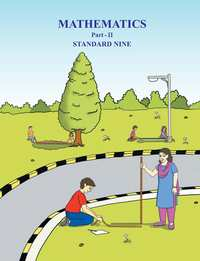## Chapter 2: Parallel Lines

Practice Set 2.1Practice Set 2.2Problem Set 2

### Balbharati solutions for Maharashtra state board SSC 9th Standard Maths 2 Geometry Chapter 2 Parallel Lines Practice Set 2.1 [Pages 17 - 18]

Practice Set 2.1 | Q 1 | Page 17

In the given figure, line RP || line MS and line DK is their transversal. ∠ DHP = 85°.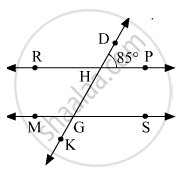Find the measures of the following angles.
(i) ∠ RHD
(ii) ∠ PHG
(iii) ∠HGS
(iv) ∠MGK
Practice Set 2.1 | Q 2 | Page 17

In the given figure, line p || line q and line l and line m are transversals. Measures of some angles are shown.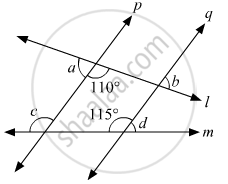Hence find the measures of anglea, angle b, angle c, angled.

Practice Set 2.1 | Q 3 | Page 17

In the given figure, line l || line m and line n || line p.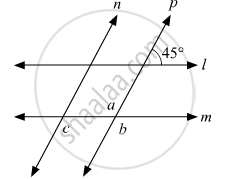Find anglea, angle b, angle c  from the given measure of an angle.

Practice Set 2.1 | Q 4 | Page 17

In the given figure, sides of angle PQR and angle XYZ are parallel to each other.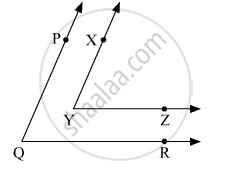Prove that, angle PQR  ≅ angle XYZ.

Practice Set 2.1 | Q 5 | Page 18

In the given figure, line AB || line CD and  line PQ is transversal. Measure of one of the angles is given. Hence find the measures of the following angles.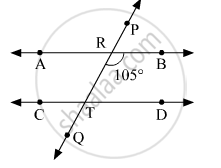(i) ∠ART
(ii) ∠CTQ
(iii) ∠DTQ
(iv) ∠PRB

### Balbharati solutions for Maharashtra state board SSC 9th Standard Maths 2 Geometry Chapter 2 Parallel Lines Practice Set 2.2 [Pages 21 - 22]

Practice Set 2.2 | Q 1 | Page 21

In the given figure, y = 108° and x = 71° Are the lines m and n parallel ? Justify ?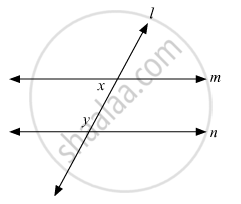Practice Set 2.2 | Q 2 | Page 21

In the given figure, if angle a ≅ angle b then prove that line l || line m.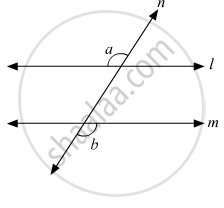Practice Set 2.2 | Q 3 | Page 21

In the given figure, if angle a ≅ angle b and angle x ≅ angle y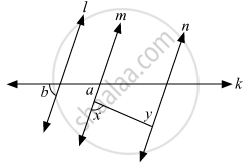then prove that line l || line n.

Practice Set 2.2 | Q 4 | Page 21

In the given figure, if ray BA || ray DE, angle C = 50° and angle D = 100° . Find the measure of angle ABC.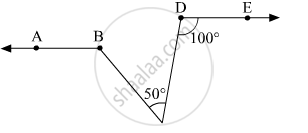Practice Set 2.2 | Q 5 | Page 22

In the given figure, ray AE || ray BD, ray AF is the bisector of angle EAB and ray BC is the bisector of angle ABD.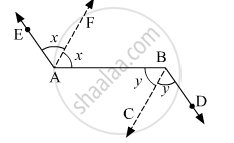Prove that line AF || line BC.

Practice Set 2.2 | Q 6 | Page 22

A transversal EF of line AB and line CD intersects the lines at point P and Q respectively. Ray PR and ray QS are parallel and bisectors angle BPQ and angle PQC respectively.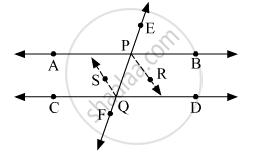Prove that line AB || line CD.

### Balbharati solutions for Maharashtra state board SSC 9th Standard Maths 2 Geometry Chapter 2 Parallel Lines Problem Set 2 [Pages 22 - 23]

Problem Set 2 | Q 1.1 | Page 22

Select the correct alternative and fill in the blank in the following statement
If a transversal intersects two parallel lines then the sum of interior angles on the same side of the transversal is ............

• 90°

• 180°

• 360°

Problem Set 2 | Q 1.2 | Page 22

Select the correct alternative and fill in the blank in the following statement.
The number of angles formed by a transversal of two lines is ............

• 2

• 4

• 8

• 16

Problem Set 2 | Q 1.3 | Page 22

Select the correct alternative and fill in the blank in the following statement.
A transversal intersects two parallel lines. If the measure of one of the angles is 40 then the measure of its corresponding angle is .............

• 40°

• 140°

• 50°

• 180°

Problem Set 2 | Q 1.4 | Page 22

Select the correct alternative and fill in the blank in the following statement.

In ΔABC, ∠A = 76°, ∠B = 48°, ∴ C = ..............

• 66°

• 56°

• 124°

• 28°

Problem Set 2 | Q 1.5 | Page 22

Select the correct alternative and fill in the blank in the following statement.
Two parallel lines are intersected by a transversal. If measure of one of the alternate interior angles is 75° then the measure of the other angle is .............

• 105°

• 15°

• 75°

• 45°

Problem Set 2 | Q 2 | Page 22

Ray PQ and ray PR are perpendicular to each other. Points B and A are in the interior and exterior of angle QPR respectively. Ray PB and ray PA are perpendicular to each other.Draw a figure showing all these rays and write -
(i) A pair of complementary angles
(ii) A pair of supplementary angles.
(iii) A pair of congruent angles.

Problem Set 2 | Q 3 | Page 23

Prove that, if a line is perpendicular to one of the two parallel lines, then it is perpendicular to the other line also.

Problem Set 2 | Q 4 | Page 23

In the given figure, measures of some angles are shown.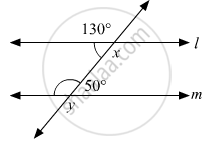Using the measures find the measures of angle x and angle y and hence show that line l || line m.

Problem Set 2 | Q 5 | Page 23

Line AB || line CD || line EF and line QP is their transversal. If y : z = 3 : 7 then find  the measure of angle x.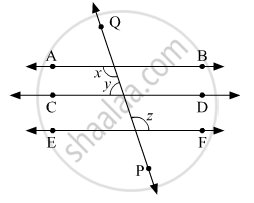Problem Set 2 | Q 6 | Page 23

In the given figure, if line q || line r, line p is their transversal and if a = 80° find the values of f and g.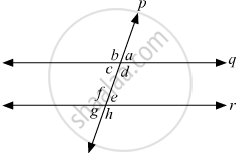Problem Set 2 | Q 7 | Page 23

In the given figure, if line AB || line CF  and line BC || line ED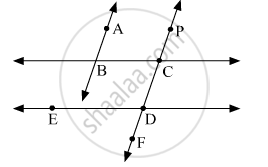then prove that angle ABC = angle FDE.

Problem Set 2 | Q 8 | Page 23

In the given figure, line PS is a transversal  of parallel line AB and line CD. If Ray QX, ray QY, ray RX, ray RY are angle bisectors, then prove that square QXRY is a rectangle.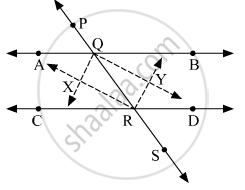## Chapter 2: Parallel Lines

Practice Set 2.1Practice Set 2.2Problem Set 2## Balbharati solutions for Maharashtra state board SSC 9th Standard Maths 2 Geometry chapter 2 - Parallel Lines

Balbharati solutions for Maharashtra state board SSC 9th Standard Maths 2 Geometry chapter 2 (Parallel Lines) include all questions with solution and detail explanation. This will clear students doubts about any question and improve application skills while preparing for board exams. The detailed, step-by-step solutions will help you understand the concepts better and clear your confusions, if any. Shaalaa.com has the Maharashtra State Board Maharashtra state board SSC 9th Standard Maths 2 Geometry solutions in a manner that help students grasp basic concepts better and faster.

Further, we at Shaalaa.com provide such solutions so that students can prepare for written exams. Balbharati textbook solutions can be a core help for self-study and acts as a perfect self-help guidance for students.

Concepts covered in Maharashtra state board SSC 9th Standard Maths 2 Geometry chapter 2 Parallel Lines are Concept of Parallel Line, Concept for Properties of Parallel Lines with Transversal, Use of properties of parallel lines, Test for Parallel Line.

Using Balbharati Class 9 solutions Parallel Lines exercise by students are an easy way to prepare for the exams, as they involve solutions arranged chapter-wise also page wise. The questions involved in Balbharati Solutions are important questions that can be asked in the final exam. Maximum students of Maharashtra State Board Class 9 prefer Balbharati Textbook Solutions to score more in exam.

Get the free view of chapter 2 Parallel Lines Class 9 extra questions for Maharashtra state board SSC 9th Standard Maths 2 Geometry and can use Shaalaa.com to keep it handy for your exam preparation